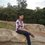Here is a very simple but interesting conjecture.

Take any natural number n>=5. If n is composite (a number having factors other than 1 and itself), add up all of its prime factors. If n is prime (a number having only two factors: 1 and the number itself), just add one to it. Repeat the process indefinitely. The conjecture states that no matter what number you start with, you shall always eventually reach the 'Perfect Number 6'.

Can anybody prove it??Note by Piyush Gupta
6 years, 6 months ago

This discussion board is a place to discuss our Daily Challenges and the math and science related to those challenges. Explanations are more than just a solution — they should explain the steps and thinking strategies that you used to obtain the solution. Comments should further the discussion of math and science.

When posting on Brilliant:

• Use the emojis to react to an explanation, whether you're congratulating a job well done , or just really confused .
• Ask specific questions about the challenge or the steps in somebody's explanation. Well-posed questions can add a lot to the discussion, but posting "I don't understand!" doesn't help anyone.
• Try to contribute something new to the discussion, whether it is an extension, generalization or other idea related to the challenge.

MarkdownAppears as
*italics* or _italics_ italics
**bold** or __bold__ bold
- bulleted- list
• bulleted
• list
1. numbered2. list
1. numbered
2. list
Note: you must add a full line of space before and after lists for them to show up correctly
paragraph 1paragraph 2

paragraph 1

paragraph 2

[example link](https://brilliant.org)example link
> This is a quote
This is a quote
    # I indented these lines
# 4 spaces, and now they show
# up as a code block.

print "hello world"
# I indented these lines
# 4 spaces, and now they show
# up as a code block.

print "hello world"
MathAppears as
Remember to wrap math in $$ ... $$ or $ ... $ to ensure proper formatting.
2 \times 3 $2 \times 3$
2^{34} $2^{34}$
a_{i-1} $a_{i-1}$
\frac{2}{3} $\frac{2}{3}$
\sqrt{2} $\sqrt{2}$
\sum_{i=1}^3 $\sum_{i=1}^3$
\sin \theta $\sin \theta$
\boxed{123} $\boxed{123}$

Sort by:

Let $f(n)$ be the sum of the prime factors of $n$ if $n$ is composite and $n+1$ if $n$ is prime.

We must show that the sequence $n, f(n), f(f(n)), f(f(f(n))), \ldots$ has $6$ in it.

Note that $f(5) = 6$, $6 = 6$, and $f(f(7)) = f(8) = 6$, Clearly, the conjecture holds for $5 \le n \le 7$.

.

If $n \ge 8$ is composite, let $n = \prod p_i$ for some primes $p_i$ not necessarily distinct. Then $f(n) = \sum p_i$.

If $f(n) < 5$, then we must have the sum of two or more primes less than $5$.

The only possibility is $2+2 = 4$, but then $n = 2 \cdot 2 = 4 < 8$, a contradiction. Therefore, $f(n) \ge 5$.

Also, for each $i$, we have $p_i \le \dfrac{n}{2}$. So, $\dfrac{\ln p_i}{p_i} \ge \dfrac{\ln (n/2)}{(n/2)}$, and thus, $\ln n = \sum \ln p_i \ge \dfrac{\ln (n/2)}{(n/2)} \sum p_i$.

Therefore, $f(n) = \sum p_i \le \dfrac{n}{2} \dfrac{\ln n}{\ln(n/2)} = \dfrac{n}{2} \dfrac{\ln n}{\ln n - \ln 2} = \dfrac{n}{2} \dfrac{1}{1 - \tfrac{\ln 2}{\ln n}} \le \dfrac{n}{2} \cdot \dfrac{3}{2} = \dfrac{3}{4}n \le n-2$.

Hence, if $n \ge 8$ is composite, we have $5 \le f(n) \le n-2$.

.

If $n \ge 8$ is prime, then $n+1 \ge 8$ is composite. Therefore, $5 \le f(f(n)) = f(n+1) \le n-1$.

.

Therefore, if $n \ge 8$, we have either $5 \le f(n) \le n-2$ or $5 \le f(f(n)) \le n-1$.

So, by principle of infinite descent, we have $5 \le f^{(k)}(n) \le 7$ for some finite positive integer $k$.

Then, since $f(5) = 6$, $6 = 6$, and $f(f(7)) = f(8) = 6$, the conjecture holds for all $n \ge 8$.

Therefore, the conjecture holds for all $n \ge 5$ as desired.

- 6 years, 6 months ago

This reminds me of the mystery of the number 276. Find the sum of all of the proper divisors of 276, and then the sum of the proper divisors of that number, and repeat. Does this sequence ever settle down or become periodic? For perfect numbers, this sequence just repeats: s(6)=1+2+3=6...

- 6 years, 6 months ago

Of course, it is much harder (if not impossible) to show that $n, s(n), s(s(n)), \ldots$ eventually has a term less than $n$. This makes that problem (as well as the Collatz Conjecture) difficult to solve.

- 6 years, 6 months ago

I don't understand..i took 8, only prime factor is 2, i didn't end up in 6? am i supposed to add 2 three times since $2^{3} = 8$?

- 6 years, 6 months ago

Yeah, $8$ has three prime factors $2$.

- 6 years, 6 months ago